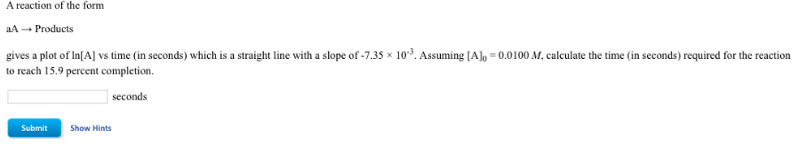# Problem: A reaction of the form aA → Products gives a plot of In[A] vs time (in seconds) which is a straight line with a slope of -7.35 x 103. Assuming [A]o = 0.0100 M, calculate the time (in seconds) required for the reaction to reach 15.9 percent completion.

###### FREE Expert Solution
86% (159 ratings)###### Problem Details

A reaction of the form

aA → Products

gives a plot of In[A] vs time (in seconds) which is a straight line with a slope of -7.35 x 103. Assuming [A]o = 0.0100 M, calculate the time (in seconds) required for the reaction to reach 15.9 percent completion.